# An RC circuit as shown in the figure is driven by a AC source generating a square wave.

Question:

An $\mathrm{RC}$ circuit as shown in the figure is driven by a $\mathrm{AC}$ source generating a square wave. The output wave pattern monitored by CRO would look close to :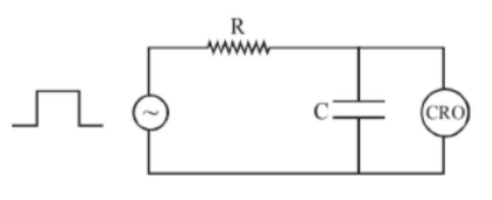1.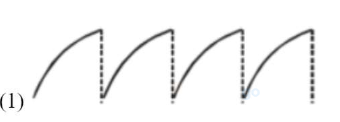2.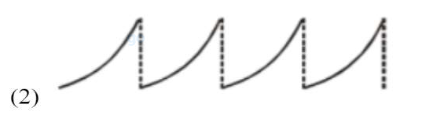3.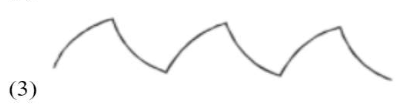4.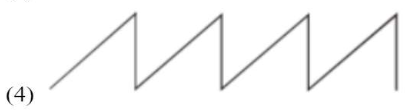Correct Option: 3,

Solution: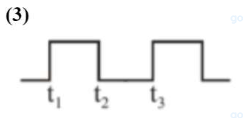For $\mathrm{t}_{1}-\mathrm{t}_{2}$ Charging graph $\mathrm{t}_{2}-\mathrm{t}_{3}$ Discharging graph